# High School Math : How to find the surface area of a prism

## Example Questions

### Example Question #601 : Problem Solving

Angie is painting a 2 foot cube for a play she is in. She needs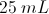of paint for every square foot she paints. How much paint does she need?

It is impossible to convert between metric units and feet.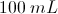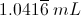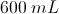Explanation:

First we must calculate the surface area of the cube. We know that there are six surfaces and each surface has the same area: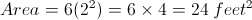Now we will determine the amount of paint needed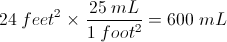### Example Question #2 : How To Find The Surface Area Of A Prism

Find the surface area of the following triangular prism.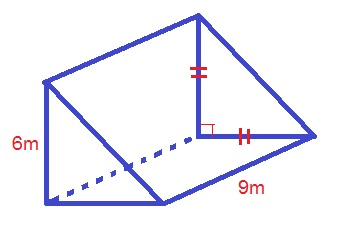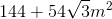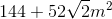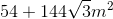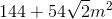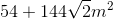Explanation:

The formula for the surface area of a triangular prism is: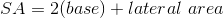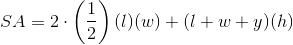Whereis the length of the triangle,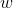is the width of the triangle,is the hypotenuse of the triangle, andis the height of the prism

Use the formula for a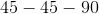triangle to solve for the length of the hypotenuse: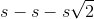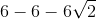Plugging in our values, we get: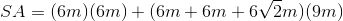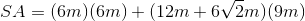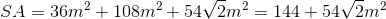### Example Question #1 : How To Find The Surface Area Of A Prism

Find the surface area of the following triangular prism.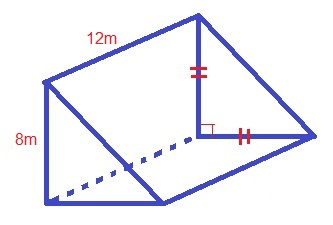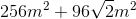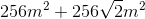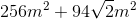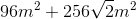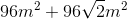Explanation:

The formula for the surface area of a triangular prism is: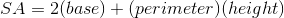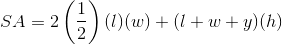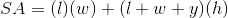Whereis the length of the base,is the width of the base,is the hypotenuse of the base, andis the height of the prism

Use the formula for atriangle to find the length of the hypotenuse: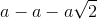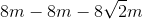Plugging in our values, we get: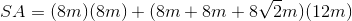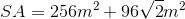### Example Question #4 : How To Find The Surface Area Of A Prism

Find the surface area of the following triangular prism.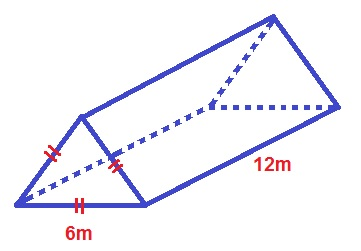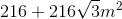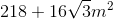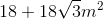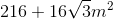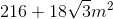Explanation:

The formula for the surface area of an equilateral, triangular prism is: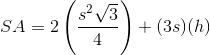Whereis the length of the triangle side andis the length of the height.

Plugging in our values, we get: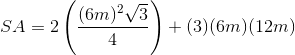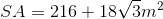### Example Question #1 : How To Find The Surface Area Of A Prism

David wants to paint the walls in his bedroom. The floor is covered by a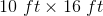carpet.  The ceiling is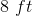tall. He selects a paint that will cover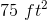per quart and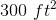per gallon. How much paint should he buy?

1 gallon and 1 quart

3 quarts

1 gallon

2 gallons and 1 quart

1 gallon and 2 quarts

1 gallon and 2 quarts

Explanation:

Find the surface area of the walls: SAwalls = 2lh + 2wh, where the height is 8 ft, the width is 10 ft, and the length is 16 ft.

This gives a total surface area of 416 ft2. One gallon covers 300 ft2, and each quart covers 75 ft2, so we need 1 gallon and 2 quarts of paint to cover the walls.

### Example Question #1 : How To Find The Surface Area Of A Prism

A box is 5 inches long, 5 inches wide, and 4 inches tall. What is the surface area of the box?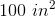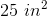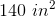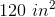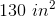Explanation:

The box will have six total faces: an identical "top and bottom," and identical "left and right," and an identical "front and back." The total surface area will be the sum of these faces.

Since the six faces consider of three sets of pairs, we can set up the equation as: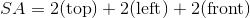Each of these faces will correspond to one pair of dimensions. Multiply the pair to get the area of the face.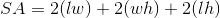Substitute the values from the question to solve.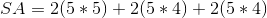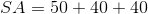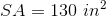### Example Question #1 : How To Find The Surface Area Of A Prism

What is the surface area of a rectangular brick with a length of 12 in, a width of 8 in, and a height of 6 in?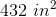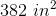None of the answers are correct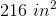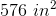Explanation:

The formula for the surface area of a rectangular prism is given by:

SA = 2LW + 2WH + 2HL

SA = 2(12 * 8) + 2(8 * 6) + 2(6 * 12)

SA = 2(96) + 2(48) + 2(72)

SA = 192 + 96 + 144

SA = 432 in2

216 in2  is the wrong answer because it is off by a factor of 2

576 in3 is actually the volume, V = L * W * H

### Example Question #8 : How To Find The Surface Area Of A Prism

What is the surface area of an equilateral triangluar prism with edges of 6 in and a height of 12 in?

Let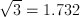and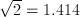.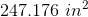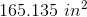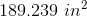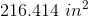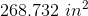Explanation:

The surface area of the prism can be broken into three rectangular sides and two equilateral triangular bases.

The area of the sides is given by: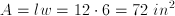, so for all three sides we get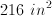.

The equilateral triangle is also an equiangular triangle by definition, so the base has congruent sides of 6 in and three angles of 60 degrees.  We use a special right traingle to figure out the height of the triangle: 30 - 60 - 90.  The height is the side opposite the 60 degree angle, so it becomes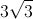or 5.196.

The area for a triangle is given by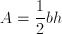and since we need two of them we get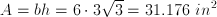.

Therefore the total surface area is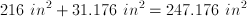.